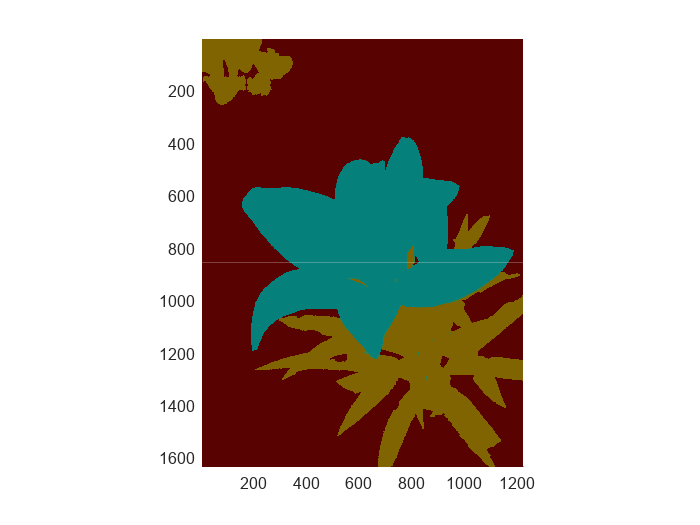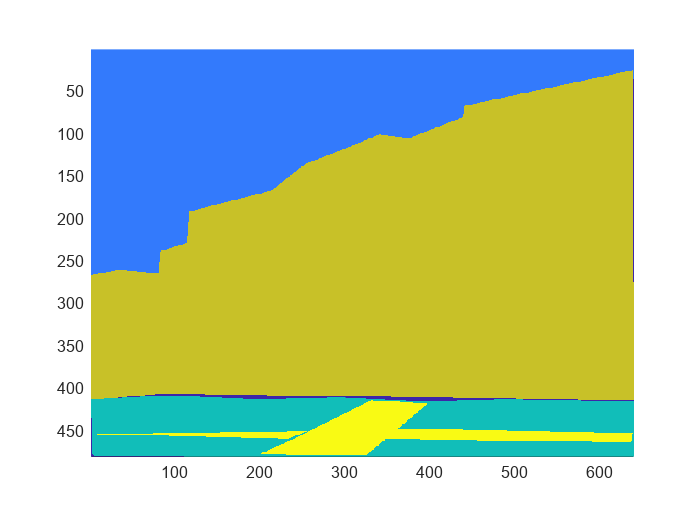# countEachLabel

Counts number of pixel labels for each class

Since R2021a

## Syntax

``counts = countEachLabel(bimds)``
``counts = countEachLabel(___,Name,Value)``

## Description

example

````counts = countEachLabel(bimds)` counts the occurrence of each pixel label in all the blocks represented by the blocked image datastore `bimds`.```

example

````counts = countEachLabel(___,Name,Value)` specifies additional name-value arguments.If `bimds` contains categorical data, `countEachLabel` obtains the class names from the categories specified in the `InitialValue` property of the first blocked image. In this case, do not specify the `Classes` and `PixelLabelIDs` name-value arguments. If `bimds` contains numeric data, you must provide values for the `Classes` and `PixelLabelIDs` name-value arguments.```

## Examples

collapse all

Create a blocked image from a sample label image.

```bim = blockedImage("yellowlily-segmented.png",BlockSize=[512 512]); b = bigimageshow(bim); showlabels(b,bim)```Create a blocked image datastore from the blocked image.

`bimds = blockedImageDatastore(bim);`

Count the labels in the blocked image datastore. Labels 0 and 3 both map to `"Background"`.

```countEachLabel(bimds, ... Classes=["Background" "Flower" "Leaf" "Background"], ... PixelLabelIDs=[0 1 2 3])```
```ans=3×3 table Name PixelCount BlockPixelCount ____________ __________ _______________ "Background" 2.3706e+06 3.1457e+06 "Flower" 4.3349e+05 1.5729e+06 "Leaf" 3.4159e+05 2.0972e+06 ```

`load("buildingPixelLabeled.mat");`

Create a `blockedImage` to manage the pixel label data.

```blockedLabeledImage = blockedImage(label,BlockSize=[200 150]); bigimageshow(blockedLabeledImage)```Create a `blockedImageDatastore` that reads blocks of size 200-by-150 pixels at the finest resolution level from `blockedLabeledImage`.

`blockLabelDS = blockedImageDatastore(blockedLabeledImage);`

Count the number of pixel labels for each class.

`tbl = countEachLabel(blockLabelDS)`
```tbl=4×3 table Name PixelCount BlockPixelCount __________ __________ _______________ "building" 1.8036e+05 4.5e+05 "grass" 32983 1.5e+05 "sidewalk" 10491 1.5e+05 "sky" 81525 1.8e+05 ```

Balance the classes by using uniform prior weighting.

```prior = 1/height(tbl); uniformClassWeights = prior ./ tbl.PixelCount```
```uniformClassWeights = 4×1 10-4 × 0.0139 0.0758 0.2383 0.0307 ```

Balance the classes by using inverse frequency weighting.

``` totalNumberOfPixels = sum(tbl.PixelCount); freq = tbl.PixelCount / totalNumberOfPixels; invFreqClassWeights = 1./freq```
```invFreqClassWeights = 4×1 1.6931 9.2580 29.1067 3.7456 ```

Balance the classes by using median frequency weighting.

```freq = tbl.PixelCount ./ tbl.BlockPixelCount; medFreqClassWeights = median(freq) ./ freq```
```medFreqClassWeights = 4×1 0.7743 1.4114 4.4373 0.6852 ```

## Input Arguments

collapse all

Blocked image datastore, specified as a `blockedImageDatastore` object.

### Name-Value Arguments

Specify optional pairs of arguments as `Name1=Value1,...,NameN=ValueN`, where `Name` is the argument name and `Value` is the corresponding value. Name-value arguments must appear after other arguments, but the order of the pairs does not matter.

Example: `countEachLabel(lbimds,Classes=["Background","Flower","Leaf","Background"],PixelLabelIDs=[0,1,2,3])`

Before R2021a, use commas to separate each name and value, and enclose `Name` in quotes.

Example: `countEachLabel(lbimds,"Classes",["Background","Flower","Leaf","Background"],"PixelLabelIDs",[0,1,2,3])`

Class names, specified as a string array or a cell array of character vectors.

Example: `["Background","Flower","Leaf"]`

Data Types: `char` | `string` | `cell`

Values for each label, specified as a numeric array of values with the same length as `Classes`. This name-value argument provides the mapping from numeric values to the label class.

Example: `[0 1 2 3]`

Data Types: `single` | `double` | `int8` | `int16` | `int32` | `int64` | `uint8` | `uint16` | `uint32` | `uint64`

Use new or existing parallel pool, specified as a numeric or logical `0` (`false`) or `1` (`true`). If no parallel pool is active, then `countEachLabel` opens a new pool based on the default parallel settings. This argument requires Parallel Computing Toolbox™.

Data Types: `logical`

## Output Arguments

collapse all

Counts of the occurrence of each pixel label in all blocks represented by the blocked image datastore, returned as a table that contains three variables.

Pixel Count VariablesDescription
`Name`Pixel label class name
`PixelCount`Number of pixels of a given class in all blocks
`BlockPixelCount`Total number of pixels in blocks that have an instance of the given class

## Tips

You can use the label information returned by `countEachLabel` to calculate class weights for class balancing. For example, for labeled pixel data information in `tbl`:

• Uniform class balancing weights each class such that each contains a uniform prior probability:

```numClasses = height(tbl) prior = 1/numClasses; classWeights = prior./tbl.PixelCount```

• Inverse frequency balancing weights each class such that underrepresented classes are given higher weight:

```totalNumberOfPixels = sum(tbl.PixelCount) frequency = tbl.PixelCount / totalNumberOfPixels; classWeights = 1./frequency```

• Median frequency balancing weights each class using the median frequency. The weight for each class `c` is defined as `median(imageFreq)/imageBlockFreq(c)`, where `imageBlockFreq(c)` is the number of pixels of a given class divided by the total number of pixels in image blocks that had an instance of the given class `c`.

```imageBlockFreq = tbl.PixelCount ./ tbl.BlockPixelCount classWeights = median(imageBlockFreq) ./ imageBlockFreq ```

You can pass the calculated class weights to a `pixelClassificationLayer` (Computer Vision Toolbox).

## Version History

Introduced in R2021a